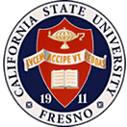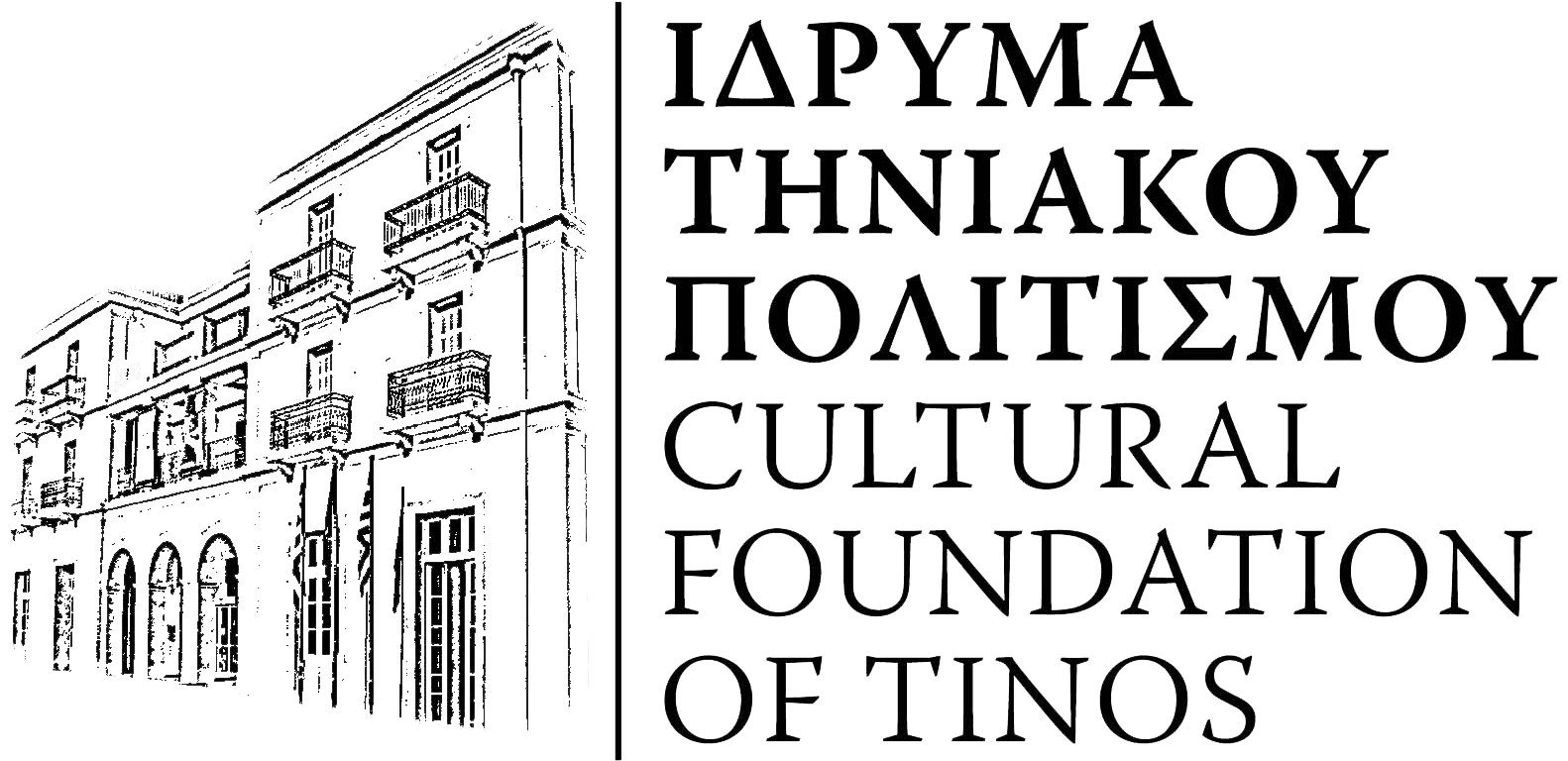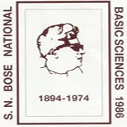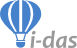## Conference topics

##### Applications

Engineering systems, Environmental systems, Operation research, Applications in medicine, Biotechnology, Logistics and planning, Medicine

##### Applied Mathematics

Fractional calculus, Numerical methods, ODE's and PDE's, Financial mathematics, Fuzzy logic, Efficient solvers, Nonlinear problems, Bifurcations, Stability, Chaos and Fractals

##### Computational Chemistry

Techniques in Computational Chemistry, Molecular vibrations, Drug design, QM/MM approach to Computational Chemistry, Molecular dynamics

##### Education

Modeling in Education

##### Evolutionary Computation

Evolutionary methods

##### Mathematical Modeling in Applications of Fundamental Physics

Weather forecast, Climate change, Earth science, Planetary science, Biophysics, Materials science, Prediction of natural phenomena, Interdisciplinary applications

##### Mathematical Modeling in Applied Physics

Semiconductor devices, Thin films, Superconductors, Organic molecules, Bioelectronics, Infrastructure design

##### Mathematical Modeling in Fundamental Physics

High-energy physics, Particle physics, Nuclear physics, Atomic physics, Molecular physics, Gravitation, Cosmology, Astrophysics, Plasma physics, Electrodynamics, Fluid dynamics, Condensed-matter physics, Chemical physics, Chaos, Statistical physics, Sociophysics

##### Modeling

Model formulation, Automation of modeling, Computer modeling, Qualitative modeling, Modular modeling and interdisciplinary modeling, Learning networks / uncertainties in modeling, Methodologies for model validation, Fitting mathematical models to real processes, Multiscale modeling, multiphysics modeling

##### Scientific Computation

Algorithms for scientific computation, Parallel algorithms and parallel computing, Grid computations, Mathematical programming, Heuristics, metaheuristics or simulation, Complex physical and technical systems, Experimental data processing, Distributed scientific computingsecretariat@icmsquare.net
(+30)6944 371 526